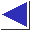# comp.lang.c FAQ list ·Question 5.3

Q: Is the abbreviated pointer comparison ``if(p)'' to test for non-null pointers valid? What if the internal representation for null pointers is nonzero?

A: It is always valid.

When C requires the Boolean value of an expression, a false value is inferred when the expression compares equal to zero, and a true value otherwise. That is, whenever one writes

```	if(expr)
```
where ``expr'' is any expression at all, the compiler essentially acts as if it had been written as
```	if((expr) != 0)
```
Substituting the trivial pointer expression ``p'' for ``expr'', we have
```	if(p)	is equivalent to	if(p != 0)
```
and this is a comparison context, so the compiler can tell that the (implicit) 0 is actually a null pointer constant, and use the correct null pointer value. There is no trickery involved here; compilers do work this way, and generate identical code for both constructs. The internal representation of a null pointer does not matter.

The boolean negation operator, !, can be described as follows:

```	!expr	is essentially equivalent to	(expr)?0:1
or to	((expr) == 0)
```
which leads to the conclusion that
```	if(!p)	is equivalent to	if(p == 0)
```

``Abbreviations'' such as if(p), though perfectly legal[footnote] , are considered by some to be bad style (and by others to be good style; see question 17.10).